## Kaggle competition overview¶

Housing costs demand a significant investment from both consumers and developers. And when it comes to planning a budget—whether personal or corporate—the last thing anyone needs is uncertainty about one of their biggets expenses. Sberbank, Russia’s oldest and largest bank, helps their customers by making predictions about realty prices so renters, developers, and lenders are more confident when they sign a lease or purchase a building.

Although the housing market is relatively stable in Russia, the country’s volatile economy makes forecasting prices as a function of apartment characteristics a unique challenge. Complex interactions between housing features such as number of bedrooms and location are enough to make pricing predictions complicated. Adding an unstable economy to the mix means Sberbank and their customers need more than simple regression models in their arsenal.

In this competition, Sberbank is challenging Kagglers to develop algorithms which use a broad spectrum of features to predict realty prices. Competitors will rely on a rich dataset that includes housing data and macroeconomic patterns. An accurate forecasting model will allow Sberbank to provide more certainty to their customers in an uncertain economy.

## Project data¶

Datasets and their description are on Kaggle and it's much safer than any other location I can offer.
Kaggle competition Data page

## Main assumptions¶

I'm interested in Sberbank strategy and in Moscow realty prices, so I'll try to learn as much as I can out of this project.

I'll try to examine most obvious features in datasets, then, i'll compare train and test sets to see, are they comparable. Also' I'll try to enhance the model by using macroeconomical features as well as cleaning data and feature engineering.

As a model, I'll use LightGBM. It's new to me and I want to learn, how to build a robust predictions using gradient boosting. I want to use ensemble, but I think, ensembling will take more time, and I'd better stick to one decision.

As a metric, I'll choose RMSLE, as it is the one set for this competition.

In :
import numpy as np
import pandas as pd
import pylab
import calendar
from datetime import datetime
from scipy import stats
import seaborn as sn
import matplotlib.pyplot as plt
%matplotlib inline

import lightgbm as lgb
from sklearn.metrics import mean_squared_error


### Part 1. Dataset and features description¶

Here I'm going to read data from csv files. There are three files:

1. train.csv: data and target variable for model training
2. test.csv: data to make prediction of target variable by trained model
3. macro.csv: additional data both for training and making prediction

Features described in file data_dictionary.txt, and description is significantly long, so I'll not copy that content here. Later I'll describe groups of columns.

In :
train_df = pd.read_csv("train.csv", parse_dates=['timestamp'])

In :
print (train_df.shape, test_df.shape, macro_df.shape)

(30471, 292) (7662, 291) (2485, 100)


I experienced some troubles with timestamp column at macro_df. It seems, no one else had such behavior, but I need to cast it to datetime64[ns] or compatible.

In :
macro_df['timestamp']=macro_df['timestamp'].apply(lambda s: pd.to_datetime(s+' 00:00:00'))
macro_df['timestamp'].dtype

Out:
dtype('<M8[ns]')
In :
train_df.head().T

Out:
0 1 2 3 4
id 1 2 3 4 5
timestamp 2011-08-20 00:00:00 2011-08-23 00:00:00 2011-08-27 00:00:00 2011-09-01 00:00:00 2011-09-05 00:00:00
full_sq 43 34 43 89 77
life_sq 27 19 29 50 77
floor 4 3 2 9 4
max_floor NaN NaN NaN NaN NaN
material NaN NaN NaN NaN NaN
build_year NaN NaN NaN NaN NaN
num_room NaN NaN NaN NaN NaN
kitch_sq NaN NaN NaN NaN NaN
state NaN NaN NaN NaN NaN
product_type Investment Investment Investment Investment Investment
sub_area Bibirevo Nagatinskij Zaton Tekstil'shhiki Mitino Basmannoe
area_m 6.40758e+06 9.58934e+06 4.80827e+06 1.25835e+07 8.39846e+06
raion_popul 155572 115352 101708 178473 108171
green_zone_part 0.189727 0.372602 0.11256 0.194703 0.0152337
indust_part 6.99893e-05 0.0496373 0.118537 0.0697534 0.0373165
children_preschool 9576 6880 5879 13087 5706
preschool_quota 5001 3119 1463 6839 3240
preschool_education_centers_raion 5 5 4 9 7
children_school 10309 7759 6207 13670 6748
school_quota 11065 6237 5580 17063 7770
school_education_centers_raion 5 8 7 10 9
school_education_centers_top_20_raion 0 0 0 0 0
hospital_beds_raion 240 229 1183 NaN 562
healthcare_centers_raion 1 1 1 1 4
university_top_20_raion 0 0 0 0 2
sport_objects_raion 7 6 5 17 25
additional_education_raion 3 1 1 6 2
culture_objects_top_25 no yes no no no
... ... ... ... ... ...
big_church_count_3000 2 1 0 1 70
church_count_3000 4 7 11 2 121
mosque_count_3000 0 0 0 0 1
leisure_count_3000 0 6 0 0 40
sport_count_3000 21 19 20 18 77
market_count_3000 1 1 6 3 5
green_part_5000 13.09 10.26 13.69 14.18 8.38
prom_part_5000 13.31 27.47 21.58 3.89 10.92
office_count_5000 29 66 43 8 689
office_sqm_5000 807385 2690465 1478160 244166 8404624
trc_count_5000 52 40 35 22 114
trc_sqm_5000 4036616 2034942 1572990 942180 3503058
cafe_count_5000 152 177 122 61 2283
cafe_sum_5000_min_price_avg 708.57 673.81 702.68 931.58 853.88
cafe_sum_5000_max_price_avg 1185.71 1148.81 1196.43 1552.63 1411.45
cafe_avg_price_5000 947.14 911.31 949.55 1242.11 1132.66
cafe_count_5000_na_price 12 9 10 4 143
cafe_count_5000_price_500 39 49 29 7 566
cafe_count_5000_price_1000 48 65 45 21 578
cafe_count_5000_price_1500 40 36 25 15 552
cafe_count_5000_price_2500 9 15 10 11 319
cafe_count_5000_price_4000 4 3 3 2 108
cafe_count_5000_price_high 0 0 0 1 17
big_church_count_5000 13 15 11 4 135
church_count_5000 22 29 27 4 236
mosque_count_5000 1 1 0 0 2
leisure_count_5000 0 10 4 0 91
sport_count_5000 52 66 67 26 195
market_count_5000 4 14 10 3 14
price_doc 5850000 6000000 5700000 13100000 16331452

292 rows × 5 columns

train_df is dataframe containing 292 features. Data exploratory analysis will come later. Now only brief explanations of these features, as they described in annotation to competition:

price_doc: sale price (this is the target variable)

In :
test_df.head().T

Out:
0 1 2 3 4
id 30474 30475 30476 30477 30478
timestamp 2015-07-01 00:00:00 2015-07-01 00:00:00 2015-07-01 00:00:00 2015-07-01 00:00:00 2015-07-01 00:00:00
full_sq 39 79.2 40.5 62.8 40
life_sq 20.7 NaN 25.1 36 40
floor 2 8 3 17 17
max_floor 9 17 5 17 17
material 1 1 2 1 1
build_year 1998 0 1960 2016 0
num_room 1 3 2 2 1
kitch_sq 8.9 1 4.8 62.8 1
state 3 1 2 3 1
product_type Investment OwnerOccupier Investment OwnerOccupier OwnerOccupier
sub_area Juzhnoe Butovo Poselenie Vnukovskoe Perovo Poselenie Voskresenskoe Poselenie Vnukovskoe
area_m 2.61551e+07 2.55363e+07 9.94634e+06 2.14941e+07 2.55363e+07
raion_popul 178264 4001 139322 7122 4001
green_zone_part 0.137846 0.496315 0.0654088 0.262459 0.496315
indust_part 0.0411164 0.00712232 0.225825 0.0176471 0.00712232
children_preschool 14080 275 6400 489 275
preschool_quota 11926 NaN 2232 NaN NaN
preschool_education_centers_raion 11 0 7 0 0
children_school 14892 264 6558 469 264
school_quota 24750 NaN 7966 NaN NaN
school_education_centers_raion 13 0 7 0 0
school_education_centers_top_20_raion 1 0 0 0 0
hospital_beds_raion NaN NaN 1548 NaN NaN
healthcare_centers_raion 1 0 3 0 0
university_top_20_raion 0 0 0 0 0
sport_objects_raion 13 0 13 0 0
additional_education_raion 4 0 0 2 0
culture_objects_top_25 no no no no no
... ... ... ... ... ...
cafe_count_3000_price_high 0 0 0 0 0
big_church_count_3000 1 1 2 0 1
church_count_3000 3 5 3 4 4
mosque_count_3000 1 0 0 0 0
leisure_count_3000 0 0 5 0 0
sport_count_3000 7 7 22 0 6
market_count_3000 0 0 4 0 0
green_part_5000 21.58 39.1 25.62 24.25 35.62
prom_part_5000 4.69 7.7 13.59 1.66 6.96
office_count_5000 1 2 27 0 1
office_sqm_5000 37550 177300 427889 0 117300
trc_count_5000 8 6 26 0 4
trc_sqm_5000 299166 231300 1024431 0 201300
cafe_count_5000 19 20 179 5 20
cafe_sum_5000_min_price_avg 676.47 733.33 668.97 1560 747.37
cafe_sum_5000_max_price_avg 1088.24 1250 1132.18 2500 1263.16
cafe_avg_price_5000 882.35 991.67 900.57 2030 1005.26
cafe_count_5000_na_price 2 2 5 0 1
cafe_count_5000_price_500 5 4 53 1 4
cafe_count_5000_price_1000 4 8 64 0 8
cafe_count_5000_price_1500 8 4 42 1 5
cafe_count_5000_price_2500 0 1 11 1 1
cafe_count_5000_price_4000 0 1 4 2 1
cafe_count_5000_price_high 0 0 0 0 0
big_church_count_5000 1 2 10 0 2
church_count_5000 10 11 21 10 12
mosque_count_5000 1 0 0 0 0
leisure_count_5000 0 1 10 0 1
sport_count_5000 14 12 71 2 11
market_count_5000 1 1 11 0 1

291 rows × 5 columns

Same columns, as in train_df, but without a target.

In :
macro_df.head().T

Out:
0 1 2 3 4
timestamp 2010-01-01 00:00:00 2010-01-02 00:00:00 2010-01-03 00:00:00 2010-01-04 00:00:00 2010-01-05 00:00:00
oil_urals 76.1 76.1 76.1 76.1 76.1
gdp_quart NaN NaN NaN NaN NaN
gdp_quart_growth NaN NaN NaN NaN NaN
cpi NaN NaN NaN NaN NaN
ppi NaN NaN NaN NaN NaN
gdp_deflator NaN NaN NaN NaN NaN
balance_trade NaN NaN NaN NaN NaN
balance_trade_growth NaN NaN NaN NaN NaN
usdrub NaN NaN NaN 29.905 29.836
eurrub NaN NaN NaN 43.4054 42.96
brent NaN NaN NaN 80.12 80.59
net_capital_export NaN NaN NaN NaN NaN
gdp_annual 38807.2 38807.2 38807.2 38807.2 38807.2
gdp_annual_growth -0.0782086 -0.0782086 -0.0782086 -0.0782086 -0.0782086
average_provision_of_build_contract 5 5 5 5 5
average_provision_of_build_contract_moscow NaN NaN NaN NaN NaN
rts NaN NaN NaN NaN NaN
micex NaN NaN NaN NaN NaN
micex_rgbi_tr NaN NaN NaN NaN NaN
micex_cbi_tr NaN 175.15 178.66 183.44 183.44
deposits_value 7.48497e+06 7.48497e+06 7.48497e+06 7.48497e+06 7.48497e+06
deposits_growth NaN NaN NaN NaN NaN
deposits_rate NaN NaN NaN NaN NaN
mortgage_value 142968 142968 142968 142968 142968
mortgage_growth NaN NaN NaN NaN NaN
mortgage_rate 13.72 13.72 13.72 13.72 13.72
grp 8375.86 8375.86 8375.86 8375.86 8375.86
grp_growth NaN NaN NaN NaN NaN
income_per_cap 30789.2 30789.2 30789.2 30789.2 30789.2
... ... ... ... ... ...
rent_price_4+room_bus NaN NaN NaN NaN NaN
rent_price_3room_bus NaN NaN NaN NaN NaN
rent_price_2room_bus NaN NaN NaN NaN NaN
rent_price_1room_bus NaN NaN NaN NaN NaN
rent_price_3room_eco NaN NaN NaN NaN NaN
rent_price_2room_eco NaN NaN NaN NaN NaN
rent_price_1room_eco NaN NaN NaN NaN NaN
load_of_teachers_preschool_per_teacher 721.478 721.478 721.478 721.478 721.478
child_on_acc_pre_school 45,713 45,713 45,713 45,713 45,713
load_of_teachers_school_per_teacher 1356.11 1356.11 1356.11 1356.11 1356.11
students_state_oneshift NaN NaN NaN NaN NaN
modern_education_share NaN NaN NaN NaN NaN
old_education_build_share NaN NaN NaN NaN NaN
provision_doctors 18 18 18 18 18
provision_nurse 99.4 99.4 99.4 99.4 99.4
load_on_doctors 7872.85 7872.85 7872.85 7872.85 7872.85
power_clinics 162.9 162.9 162.9 162.9 162.9
hospital_beds_available_per_cap NaN NaN NaN NaN NaN
hospital_bed_occupancy_per_year NaN NaN NaN NaN NaN
provision_retail_space_sqm NaN NaN NaN NaN NaN
provision_retail_space_modern_sqm 690 690 690 690 690
turnover_catering_per_cap 6221 6221 6221 6221 6221
theaters_viewers_per_1000_cap 527 527 527 527 527
seats_theather_rfmin_per_100000_cap 0.41 0.41 0.41 0.41 0.41
museum_visitis_per_100_cap 993 993 993 993 993
bandwidth_sports NaN NaN NaN NaN NaN
population_reg_sports_share NaN NaN NaN NaN NaN
students_reg_sports_share 63.03 63.03 63.03 63.03 63.03
apartment_build 22825 22825 22825 22825 22825
apartment_fund_sqm NaN NaN NaN NaN NaN

100 rows × 5 columns

macro_df contains 99 features related to Russia's macroeconomy and financial sector and timestamp to join to the train_df and test_df. These stats are collected daily, so 2485 days are approximately 7 years.

### Part 2. Exploratory data analysis¶

I will explore target variable price_doc. Price can be quite imbalanced, I'll apply logarithm to see, if it can looks like normal distibution.

In :
train_df['price_doc'].describe()

Out:
count    3.047100e+04
mean     7.123035e+06
std      4.780111e+06
min      1.000000e+05
25%      4.740002e+06
50%      6.274411e+06
75%      8.300000e+06
max      1.111111e+08
Name: price_doc, dtype: float64

Target description is nice. I see, 75 quartile is 8.3 million, so reasonable. But there is some huge prices, of course, realty market may contain such offers.

I'll find skewness and kurtosis

In :
print("Skewness: %f" % train_df['price_doc'].skew())
print("Kurtosis: %f" % train_df['price_doc'].kurt())

Skewness: 4.474745
Kurtosis: 44.027368

In :
train_df['price_doc_log'] = np.log1p(train_df['price_doc'])

In :
print("Skewness: %f" % train_df['price_doc_log'].skew())
print("Kurtosis: %f" % train_df['price_doc_log'].kurt())

Skewness: -0.686715
Kurtosis: 2.246654


Skewness closer to zero and kurtosis less than original of price_doc. I think, logarithmic target will be better to predict.

In :
fig,axes = plt.subplots(ncols=2)
fig.set_size_inches(20,10)

C:\Users\16704527\AppData\Local\Continuum\anaconda3\lib\site-packages\scipy\stats\stats.py:1713: FutureWarning: Using a non-tuple sequence for multidimensional indexing is deprecated; use arr[tuple(seq)] instead of arr[seq]. In the future this will be interpreted as an array index, arr[np.array(seq)], which will result either in an error or a different result.
return np.add.reduce(sorted[indexer] * weights, axis=axis) / sumval

Out:
<matplotlib.axes._subplots.AxesSubplot at 0x111fc5c0>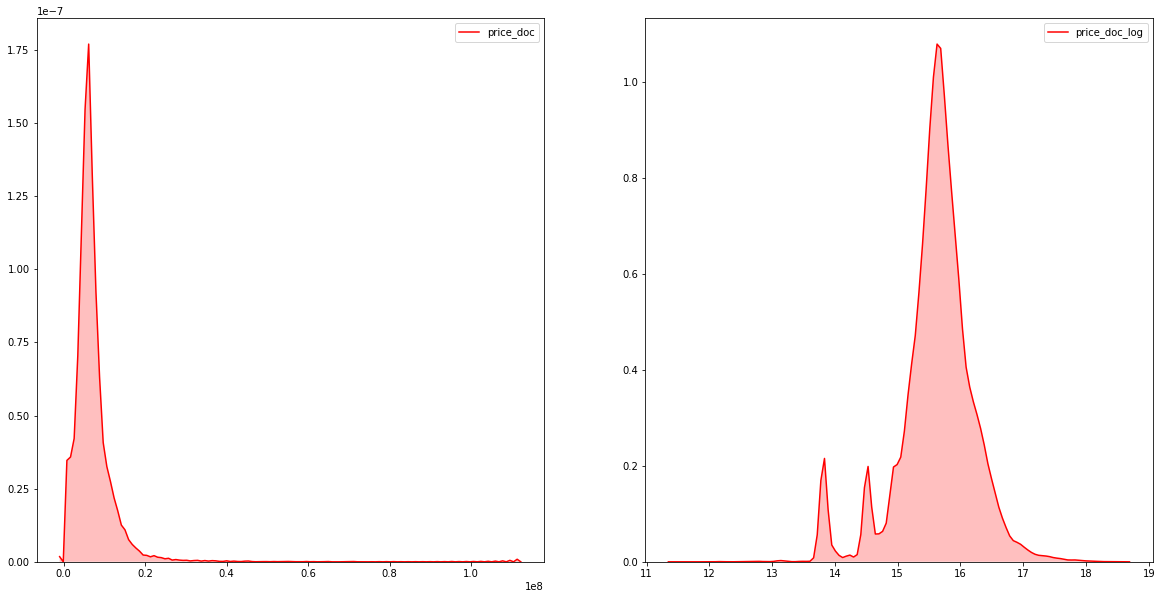In :
fig,axes = plt.subplots(ncols=2)
fig.set_size_inches(20,10)
sn.kdeplot(data=train_df[(train_df["price_doc"]>1e6) & (train_df["price_doc"]!=2e6) & (train_df["price_doc"]!=3e6)]["price_doc"], color="r", shade=True,ax=axes)
sn.kdeplot(data=train_df[(train_df["price_doc"]>1e6) & (train_df["price_doc"]!=2e6) & (train_df["price_doc"]!=3e6)]["price_doc_log"], color="r", shade=True,ax=axes)

C:\Users\16704527\AppData\Local\Continuum\anaconda3\lib\site-packages\scipy\stats\stats.py:1713: FutureWarning: Using a non-tuple sequence for multidimensional indexing is deprecated; use arr[tuple(seq)] instead of arr[seq]. In the future this will be interpreted as an array index, arr[np.array(seq)], which will result either in an error or a different result.
return np.add.reduce(sorted[indexer] * weights, axis=axis) / sumval

Out:
<matplotlib.axes._subplots.AxesSubplot at 0x113c5198>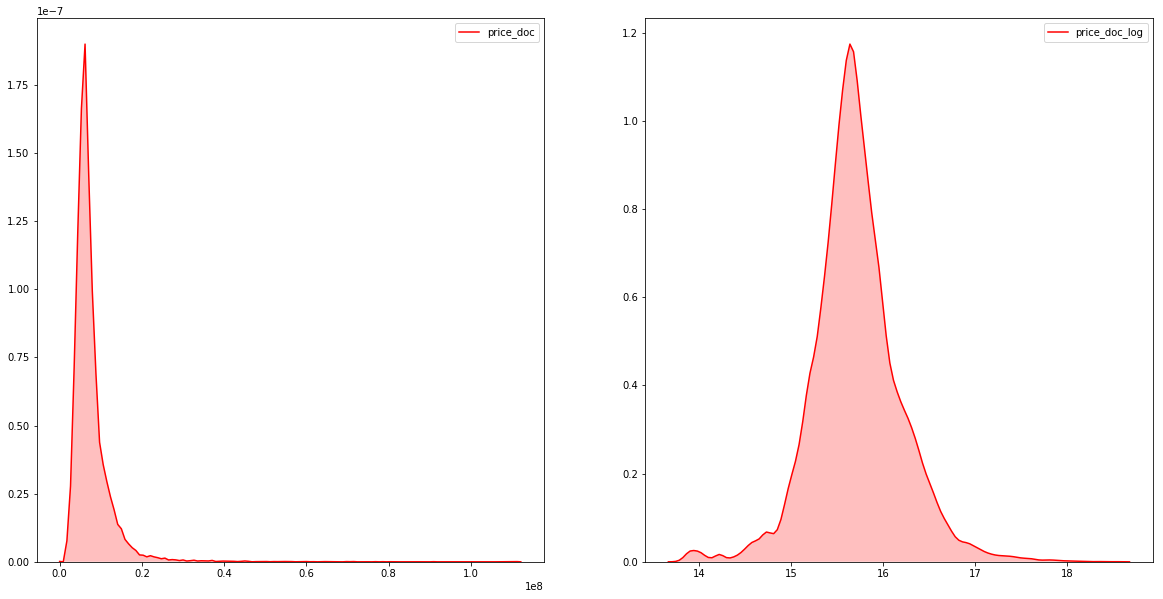Without some values, distributions seems more smooth. I'll prepare method of cleaning this values and call it at next step.

In :
def remove_abnormal_prices(df):
df.drop(df[(df["price_doc"]<=1e6) | (df["price_doc"]==2e6) | (df["price_doc"]==3e6)].index,
inplace=True)

In :
fig,axes = plt.subplots(ncols=2)
fig.set_size_inches(20, 10)
stats.probplot(train_df["price_doc_log"], dist='norm', fit=True, plot=axes)
stats.probplot(train_df[(train_df["price_doc"]>1e6) & (train_df["price_doc"]!=2e6) & (train_df["price_doc"]!=3e6)]["price_doc_log"], dist='norm', fit=True, plot=axes)

Out:
((array([-4.06125727, -3.84925825, -3.73342598, ...,  3.73342598,
3.84925825,  4.06125727]),
array([13.82049909, 13.82248716, 13.83040016, ..., 18.32709614,
18.37067606, 18.52604128])),
(0.47066126932516483, 15.709944543239672, 0.985452937433602))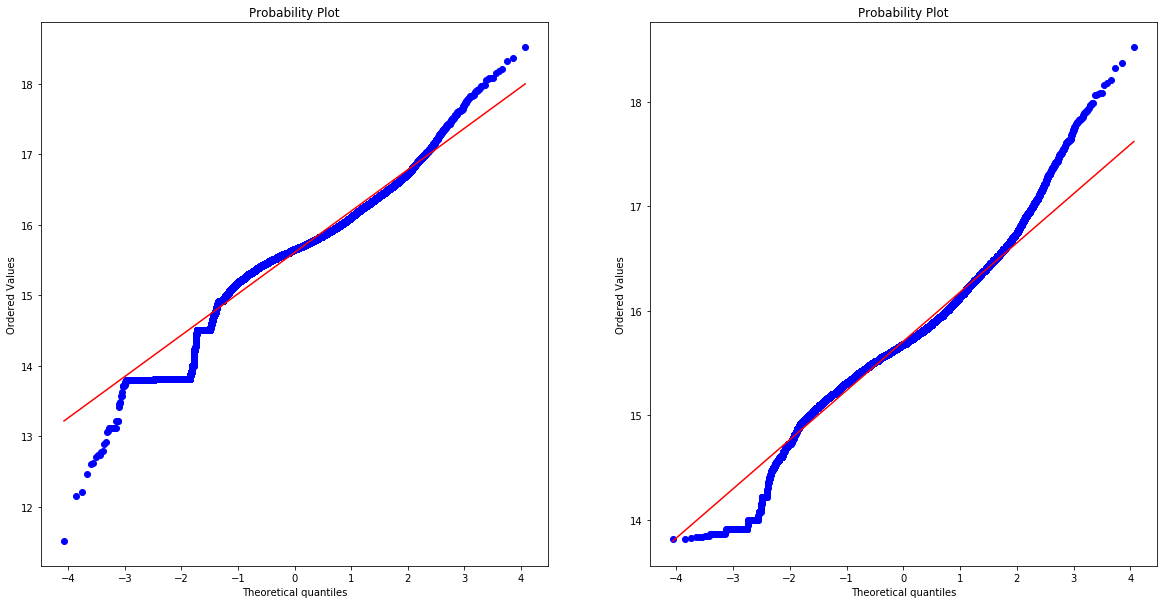It seems, logarithm target has normal distibution with some deviations. Model will be trained on log Target to get better predictions and satisfy metric condidions.

I'll look on product_type relation to price_doc_log.

In :
ax = sn.FacetGrid(train_df, col="product_type", size=6)
ax.set(ylabel='density')

C:\Users\16704527\AppData\Local\Continuum\anaconda3\lib\site-packages\seaborn\axisgrid.py:230: UserWarning: The size paramter has been renamed to height; please update your code.
warnings.warn(msg, UserWarning)
C:\Users\16704527\AppData\Local\Continuum\anaconda3\lib\site-packages\scipy\stats\stats.py:1713: FutureWarning: Using a non-tuple sequence for multidimensional indexing is deprecated; use arr[tuple(seq)] instead of arr[seq]. In the future this will be interpreted as an array index, arr[np.array(seq)], which will result either in an error or a different result.
return np.add.reduce(sorted[indexer] * weights, axis=axis) / sumval

Out:
<seaborn.axisgrid.FacetGrid at 0x114f3390>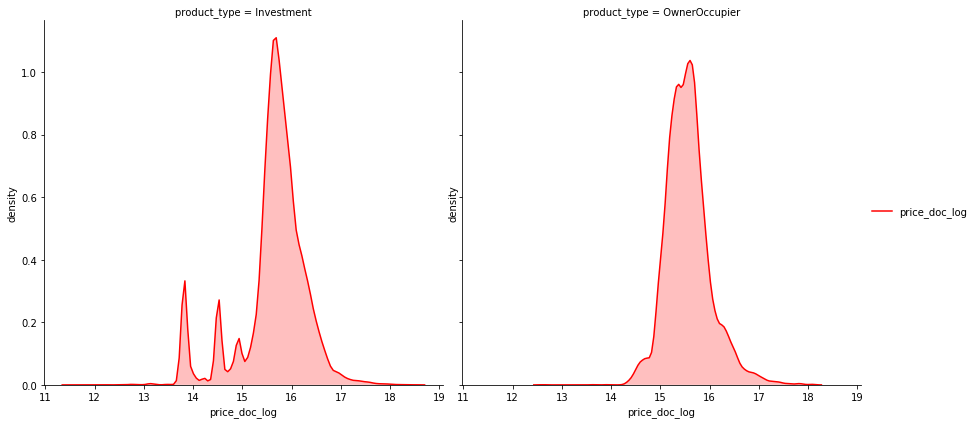Investment realty price tends to have round value, 1e6 - one million, 2e6 - two millions, etc.

### Part 3. Visual analysis of the features¶

I'll explore datatypes of features in train_df.

In :
dataTypeDf = pd.DataFrame(train_df.dtypes.value_counts()).reset_index().rename(columns={"index":"variableType",0:"count"})
fig,ax = plt.subplots()
fig.set_size_inches(20,5)
sn.barplot(data=dataTypeDf,x="variableType",y="count",ax=ax)
ax.set(xlabel='Variable Type', ylabel='Count',title="Variables Count Across Datatype")

Out:
[Text(0,0.5,'Count'),
Text(0.5,0,'Variable Type'),
Text(0.5,1,'Variables Count Across Datatype')]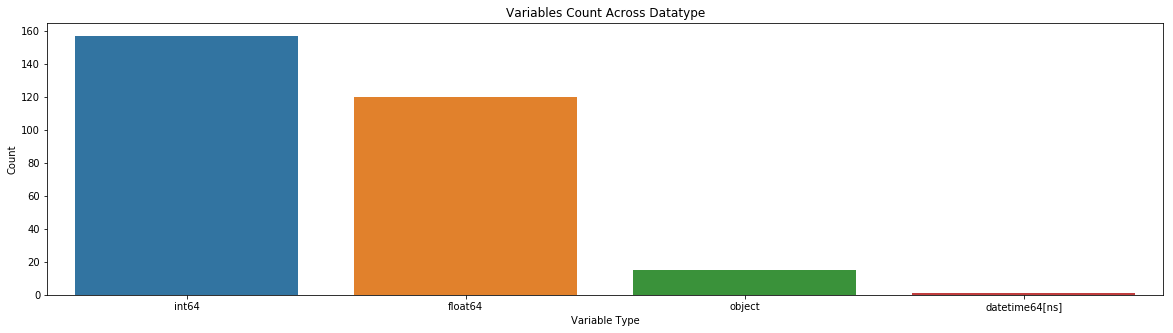int84 and float64 values are good for regression. Features marked as object are categorical variables. datetime64[ns] is timestamp column.

I'll look for missing values in train_df

In :
train_na = (train_df.isnull().sum() / len(train_df)) * 100
train_na = train_na.drop(train_na[train_na == 0].index).sort_values(ascending=False)

In :
f, ax = plt.subplots(figsize=(12, 8))
plt.xticks(rotation='90')
sn.barplot(x=train_na.index, y=train_na)
ax.set(title='Percent missing data by feature', ylabel='% missing')

Out:
[Text(0,0.5,'% missing'), Text(0.5,1,'Percent missing data by feature')]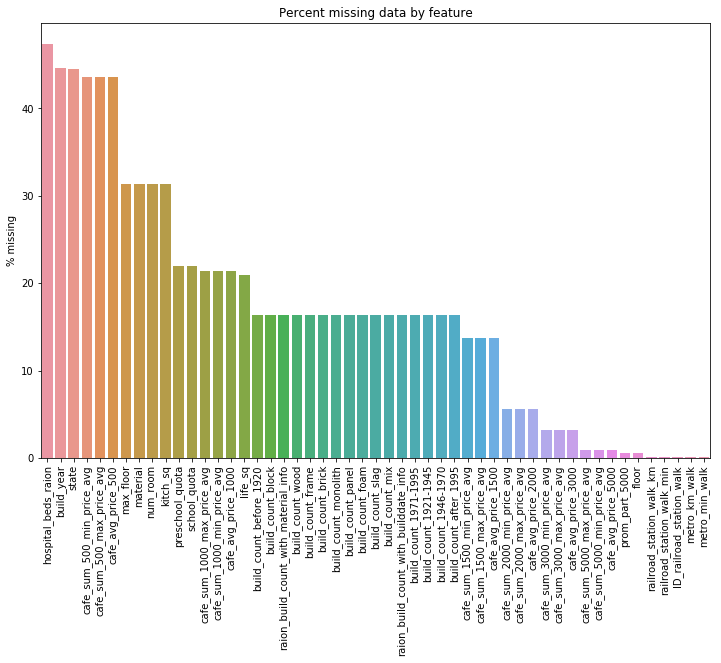Of 292 columns, 51 have missing values. The percentage of values missing ranges from 0.1% in metro_min_walk to 47.4% in hospital_beds_raion. I'll think, how to deal with it. Remain it intact is an option, though.

I need to get some new features derived from timestamp to continue.

In :
train_df['year'] = train_df['timestamp'].apply(lambda ts: ts.year)
train_df['month'] = train_df['timestamp'].apply(lambda ts:ts. month)

test_df['year'] = test_df['timestamp'].apply(lambda ts: ts.year)
test_df['month'] = test_df['timestamp'].apply(lambda ts: ts.month)

In :
fig,(ax1,ax2) = plt.subplots(ncols=2)
fig.set_size_inches(20,5)
sn.boxplot(data=train_df,y="price_doc",orient="v",ax=ax1)
sn.boxplot(data=train_df,x="price_doc",y="year",orient="h",ax=ax2)

fig1,ax3 = plt.subplots()
fig1.set_size_inches(20,5)
sn.boxplot(data=train_df,x="month",y="price_doc",orient="v",ax=ax3)
ax1.set(ylabel='Price Doc', title="Box Plot On Price Doc")
ax2.set(xlabel='Price Doc', ylabel='Year',title="Box Plot On Price Doc Across Year")
ax3.set(xlabel='Month', ylabel='Count',title="Box Plot On Price Doc Across Month")

Out:
[Text(0,0.5,'Count'),
Text(0.5,0,'Month'),
Text(0.5,1,'Box Plot On Price Doc Across Month')]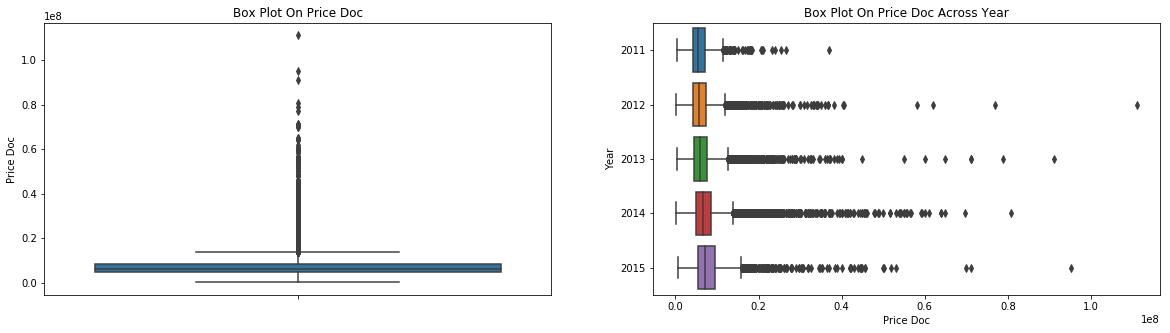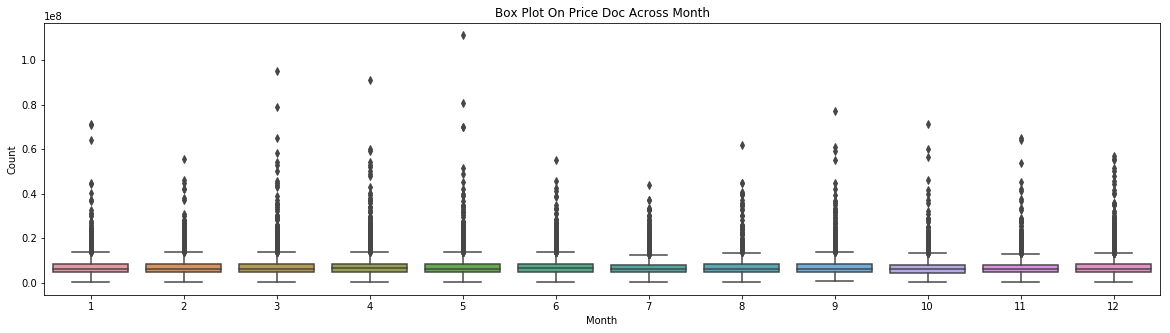In :
stateDf = pd.DataFrame(train_df[~train_df['state'].isnull()]['state'].value_counts()).reset_index().rename(columns={"index":"state","state":"count"})
stateDf
fig,ax = plt.subplots()
fig.set_size_inches(20,5)
sn.barplot(data=stateDf,x="state",y="count",ax=ax)
ax.set(xlabel='state', ylabel='Count',title="Variables Count Across Datatype")

Out:
[Text(0,0.5,'Count'),
Text(0.5,0,'state'),
Text(0.5,1,'Variables Count Across Datatype')]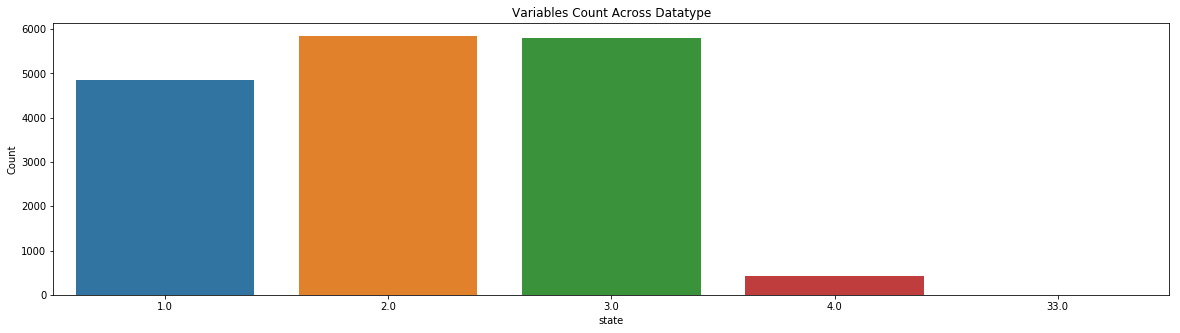State should be discrete valued between 1 and 4. There is a 33 in it that is cleary a data entry error Lets just replace it with value 3.

In :
def correct_state_33(df):
df.loc[df['state'] == 33, 'state'] = 3


I'll explore build_year feature

In :
f, ax = plt.subplots(figsize=(12, 8))
plt.xticks(rotation='90')
ind = train_df[(train_df['build_year'] <= 1691) | (train_df['build_year'] >= 2018)].index
by_df = train_df.drop(ind).sort_values(by=['build_year'])
sn.countplot(x=by_df['build_year'])
ax.set(title='Distribution of build year')

Out:
[Text(0.5,1,'Distribution of build year')]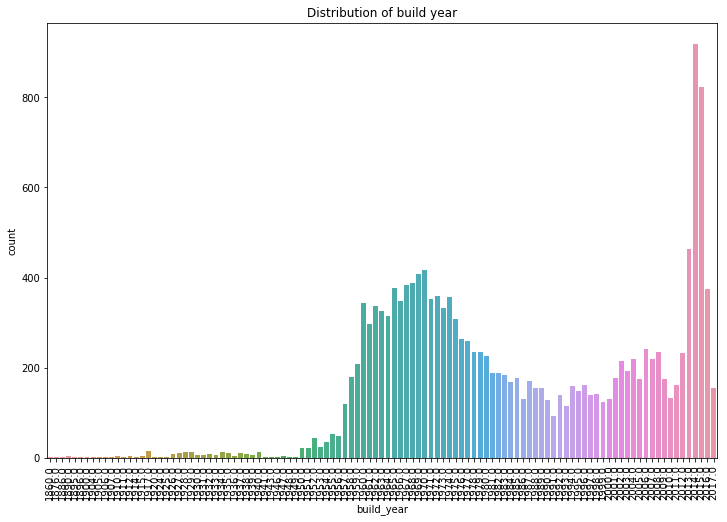The distribution appears bimodal with a peak somewhere in the early 1970s and somewhere in the past few years.

In :
buildYearDf = pd.DataFrame(train_df[(train_df['build_year']<1147)|(train_df['build_year']>2030)]['build_year'].value_counts()).reset_index().rename(columns={"index":"build_year","build_year":"count"})
buildYearDf
fig,ax = plt.subplots()
fig.set_size_inches(20,5)
sn.barplot(data=buildYearDf,x="build_year",y="count",ax=ax)
ax.set(xlabel='Build Year', ylabel='Count',title="Count Across build_year")

Out:
[Text(0,0.5,'Count'),
Text(0.5,0,'Build Year'),
Text(0.5,1,'Count Across build_year')]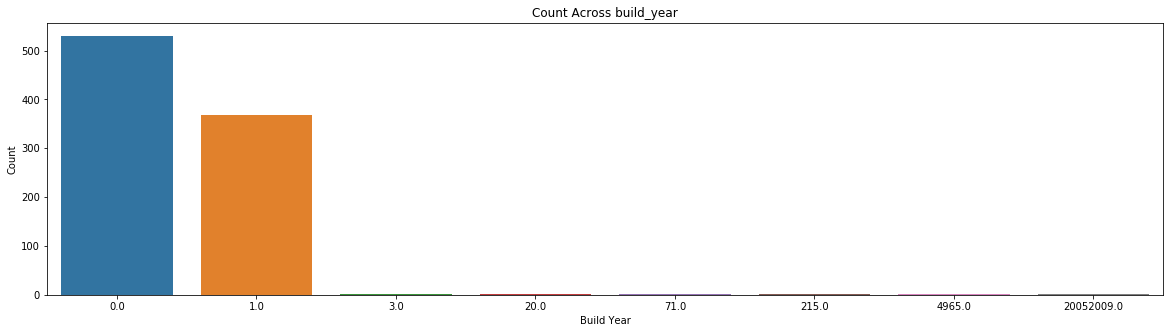Build_year has some erroneous values. Since its unclear what they should be, let's replace 20052009 with 2007, 4965 with 1965, 20 with 1920, 71 with 1971. Values 0, 1, 3 remain intact.

In :
def clean_build_year(df):
build_year_mode = df['build_year'].mode().iloc
print("build_year_mode=%s"%build_year_mode)

df.loc[df['build_year'] == 20052009, 'build_year'] = 2007
df.loc[df['build_year'] == 4965, 'build_year'] = 1965
df.loc[df['build_year'] == 20, 'build_year'] = 1920
df.loc[df['build_year'] == 71, 'build_year'] = 1971

#df.loc[df['build_year'] < 1147, 'build_year'] = build_year_mode


Now let’s see if build_year and prices are related. Here I group the data by year and take the mean of price_doc.

In :
f, ax = plt.subplots(figsize=(12, 6))
by_price = by_df.groupby('build_year')[['build_year', 'price_doc']].mean()
sn.regplot(x="build_year", y="price_doc", data=by_price, scatter=False, order=3, truncate=True)
plt.plot(by_price['build_year'], by_price['price_doc'], color='r')
ax.set(title='Mean price by year of build')

C:\Users\16704527\AppData\Local\Continuum\anaconda3\lib\site-packages\scipy\stats\stats.py:1713: FutureWarning: Using a non-tuple sequence for multidimensional indexing is deprecated; use arr[tuple(seq)] instead of arr[seq]. In the future this will be interpreted as an array index, arr[np.array(seq)], which will result either in an error or a different result.
return np.add.reduce(sorted[indexer] * weights, axis=axis) / sumval

Out:
[Text(0.5,1,'Mean price by year of build')]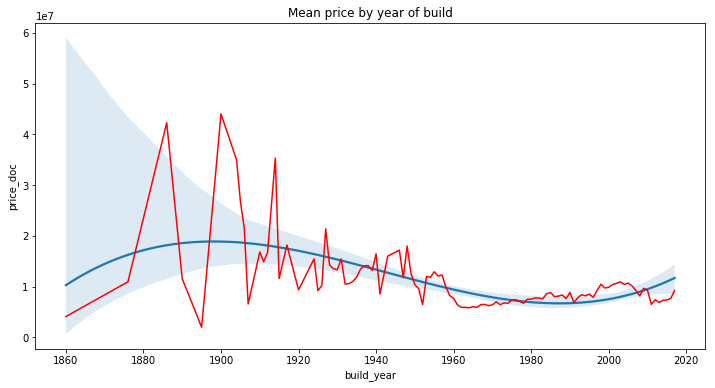The relationship appears somewhat steady over time, especially after 1960. There is some volatility in the earlier years. This is not a real effect but simply due to the sparseness of observations until around 1950.

There are internal home characteristics in the train_df. I'll build correlation matrix with column price_doc.

In :
internal_feats = ['full_sq', 'life_sq', 'floor', 'max_floor', 'build_year', 'num_room', 'kitch_sq', 'state', 'price_doc']
corrmat = train_df[internal_feats].corr()

In :
f, ax = plt.subplots(figsize=(10, 7))
plt.xticks(rotation='90')
sn.heatmap(corrmat, square=True, linewidths=.5, annot=True)

Out:
<matplotlib.axes._subplots.AxesSubplot at 0x14703940>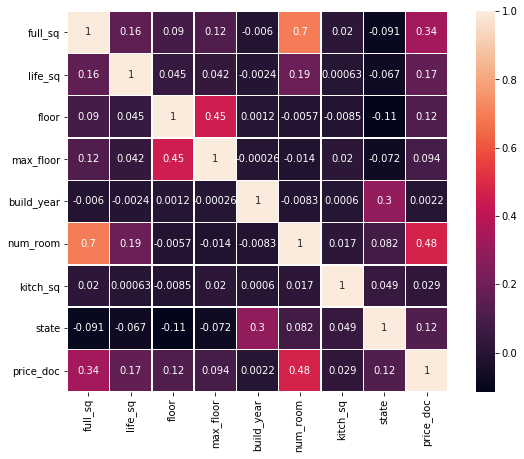Area of Home and Number of Rooms

full_sq is correlated with price. I'll take a closer look.

In :
f, ax = plt.subplots(figsize=(10, 7))
plt.scatter(x=train_df['full_sq'], y=train_df['price_doc'], c='r')

Out:
<matplotlib.collections.PathCollection at 0x189a4320>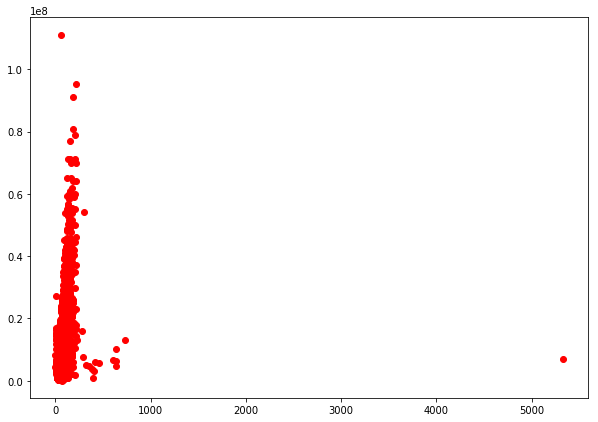There is an outlier in full_sq. Its not clear whether this is an entry error. I'll remove it.

In :
def remove_life_sq_outlier(df):
df.drop(df[df["life_sq"] > 5000].index, inplace=True)

In :
f, ax = plt.subplots(figsize=(10, 7))
ind = train_df[train_df['full_sq'] > 2000].index
plt.scatter(x=train_df.drop(ind)['full_sq'], y=train_df.drop(ind)['price_doc'], c='r', alpha=0.5)
ax.set(title='Price by area in sq meters', xlabel='Area', ylabel='Price')

Out:
[Text(0,0.5,'Price'),
Text(0.5,0,'Area'),
Text(0.5,1,'Price by area in sq meters')]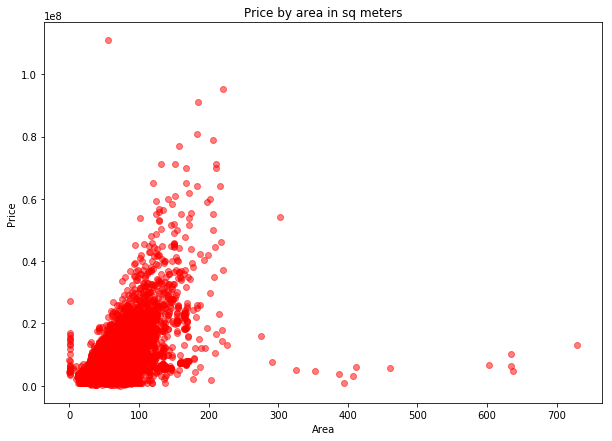The feature full_sq is defined in the data dictionary as ‘total area in square meters, including loggias, balconies and other non-residential areas’ and the life_sq is defined as ‘living area in square meters, excluding loggias, balconies and other non-residential areas.’ So it should be the case that life_sq is always less than full_sq.

In :
(train_df['life_sq'] > train_df['full_sq']).sum()

Out:
37

There are 37 observations where life_sq is greater than full_sq.

I'll explore num_room feature

In :
f, ax = plt.subplots(figsize=(10, 7))
sn.countplot(x=train_df['num_room'])
ax.set(title='Distribution of room count', xlabel='num_room')

Out:
[Text(0.5,0,'num_room'), Text(0.5,1,'Distribution of room count')]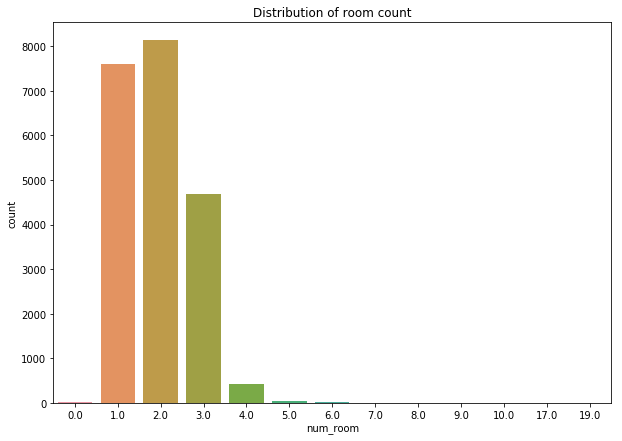A vast majority of the apartments have one, two or three rooms.

Timestamp

How does the sale price vary over the time horizon of the data set? Here I just group by the day and caclulate the median price for each day and plot it over time.

In :
f, ax = plt.subplots(figsize=(12, 6))
ts_df = train_df.groupby('timestamp')[['price_doc']].mean()
#sns.regplot(x="timestamp", y="price_doc", data=ts_df, scatter=False, truncate=True)
plt.plot(ts_df.index, ts_df['price_doc'], color='r', )
ax.set(title='Daily median price over time')

Out:
[Text(0.5,1,'Daily median price over time')]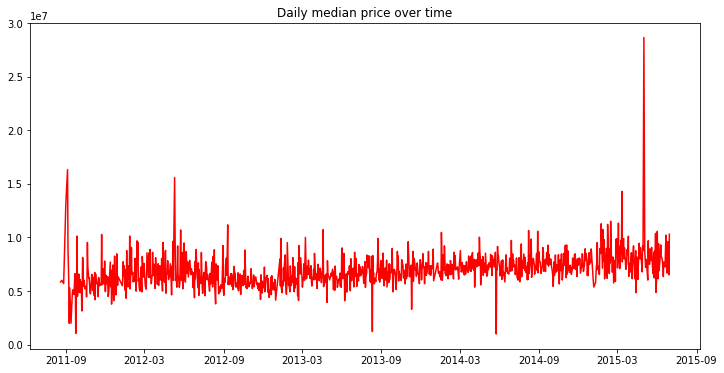And to compare with the above plot, here is the volume of sales over the same time.

In :
import datetime
import matplotlib.dates as mdates
years = mdates.YearLocator()   # every year
yearsFmt = mdates.DateFormatter('%Y')
ts_vc = train_df['timestamp'].value_counts()
f, ax = plt.subplots(figsize=(12, 6))
plt.bar(left=ts_vc.index, height=ts_vc)
ax.xaxis.set_major_locator(years)
ax.xaxis.set_major_formatter(yearsFmt)
ax.set(title='Sales volume over time', ylabel='Number of transactions')

C:\Users\16704527\AppData\Local\Continuum\anaconda3\lib\site-packages\matplotlib\__init__.py:1867: MatplotlibDeprecationWarning: The *left* kwarg to bar is deprecated use *x* instead. Support for *left* will be removed in Matplotlib 3.0
return func(ax, *args, **kwargs)

Out:
[Text(0,0.5,'Number of transactions'), Text(0.5,1,'Sales volume over time')]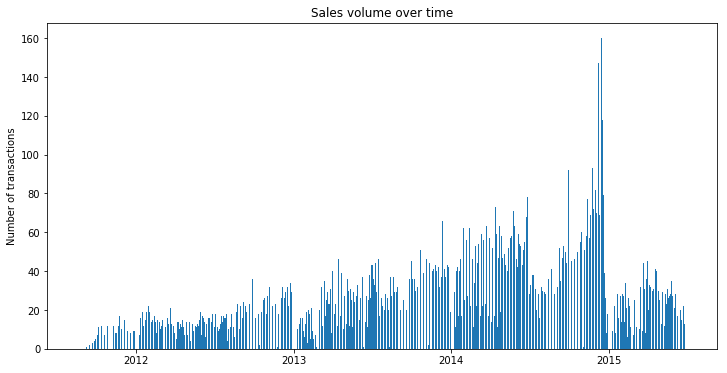Is there a seasonal component to home prices in the course of a year?

In :
f, ax = plt.subplots(figsize=(12, 8))
ts_df = train_df.groupby(by=[train_df.timestamp.dt.month])[['price_doc']].median()
plt.plot(ts_df.index, ts_df, color='r')
ax.set(title='Price by month of year')

Out:
[Text(0.5,1,'Price by month of year')]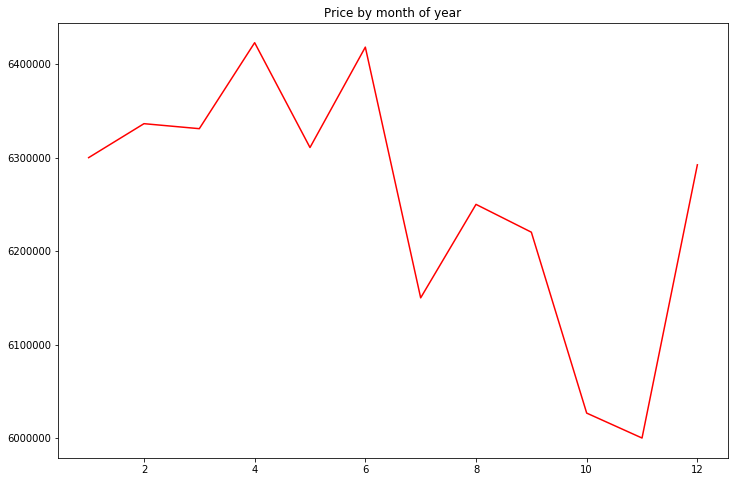Home State/Material

How do homes vary in price by condition?

In :
f, ax = plt.subplots(figsize=(12, 8))
ind = train_df[train_df['state'].isnull()].index
train_df['price_doc_log10'] = np.log10(train_df['price_doc'])
sn.violinplot(x="state", y="price_doc_log10", data=train_df.drop(ind), inner="box")
# sns.swarmplot(x="state", y="price_doc_log10", data=train_df.dropna(), color="w", alpha=.2);
ax.set(title='Log10 of median price by state of home', xlabel='state', ylabel='log10(price)')

C:\Users\16704527\AppData\Local\Continuum\anaconda3\lib\site-packages\scipy\stats\stats.py:1713: FutureWarning: Using a non-tuple sequence for multidimensional indexing is deprecated; use arr[tuple(seq)] instead of arr[seq]. In the future this will be interpreted as an array index, arr[np.array(seq)], which will result either in an error or a different result.
return np.add.reduce(sorted[indexer] * weights, axis=axis) / sumval

Out:
[Text(0,0.5,'log10(price)'),
Text(0.5,0,'state'),
Text(0.5,1,'Log10 of median price by state of home')]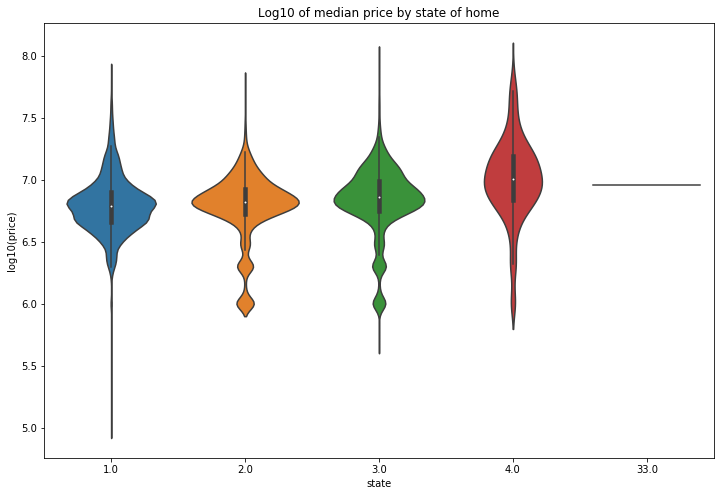It’s hard to tell from the plot, but it does appear that state 4 has the highest sale price on average. Significantly fewer homes fall under this category however. I'll check this assumption:

In :
train_df.drop(ind).groupby('state')['price_doc'].mean()

Out:
state
1.0     7.315440e+06
2.0     7.060064e+06
3.0     8.078316e+06
4.0     1.334547e+07
33.0    9.000000e+06
Name: price_doc, dtype: float64

State 4 has the highest average price by far, followed by state 3. State 1 and 2 are close.

In :
f, ax = plt.subplots(figsize=(12, 8))
ind = train_df[train_df['material'].isnull()].index
sn.violinplot(x="material", y="price_doc_log", data=train_df.drop(ind), inner="box")
# sns.swarmplot(x="state", y="price_doc_log10", data=train_df.dropna(), color="w", alpha=.2);
ax.set(title='Distribution of price by build material', xlabel='material', ylabel='log(price)')

C:\Users\16704527\AppData\Local\Continuum\anaconda3\lib\site-packages\scipy\stats\stats.py:1713: FutureWarning: Using a non-tuple sequence for multidimensional indexing is deprecated; use arr[tuple(seq)] instead of arr[seq]. In the future this will be interpreted as an array index, arr[np.array(seq)], which will result either in an error or a different result.
return np.add.reduce(sorted[indexer] * weights, axis=axis) / sumval

Out:
[Text(0,0.5,'log(price)'),
Text(0.5,0,'material'),
Text(0.5,1,'Distribution of price by build material')]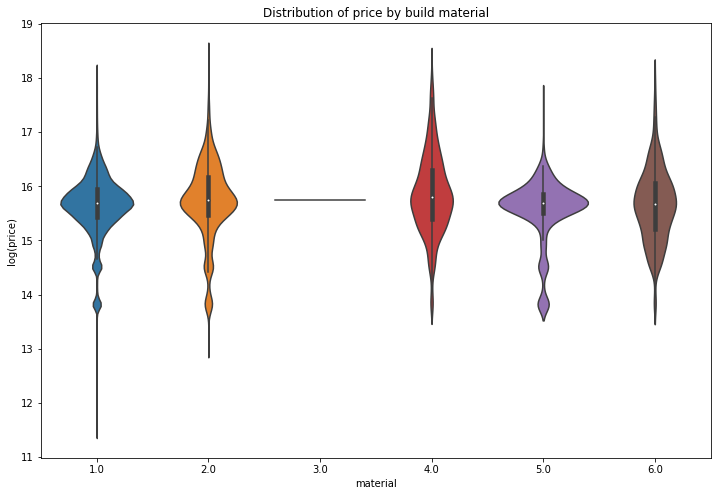It’s unclear what these values mean since this feature is not described in the data dictionary. Material 1 is by far the most common. Only one home is classifed as material 3. How does median price compare among these six materials?

In :
train_df.drop(ind).groupby('material')['price_doc'].median()

Out:
material
1.0    6500000.0
2.0    6900000.0
3.0    6931143.0
4.0    7247869.5
5.0    6492000.0
6.0    6362318.0
Name: price_doc, dtype: float64

Floor of Home

How does the floor feature compare with price? According to the correlation plot from earlier, there is a moderate positive correlation.

In :
f, ax = plt.subplots(figsize=(12, 8))
plt.scatter(x=train_df['floor'], y=train_df['price_doc_log'], c='r', alpha=0.4)
sn.regplot(x="floor", y="price_doc_log", data=train_df, scatter=False, truncate=True)
ax.set(title='Price by floor of home', xlabel='floor', ylabel='log(price)')

C:\Users\16704527\AppData\Local\Continuum\anaconda3\lib\site-packages\scipy\stats\stats.py:1713: FutureWarning: Using a non-tuple sequence for multidimensional indexing is deprecated; use arr[tuple(seq)] instead of arr[seq]. In the future this will be interpreted as an array index, arr[np.array(seq)], which will result either in an error or a different result.
return np.add.reduce(sorted[indexer] * weights, axis=axis) / sumval

Out:
[Text(0,0.5,'log(price)'),
Text(0.5,0,'floor'),
Text(0.5,1,'Price by floor of home')]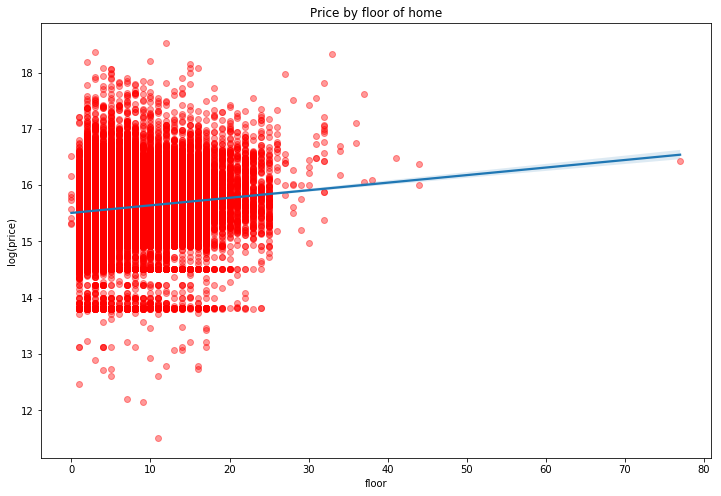On a whole, price seems to rise with the floor, although the effect is pretty small. Along the same lines, I wonder if the height of building is correlated with price. Well look at this using max_floor as a proxy for height.

In :
f, ax = plt.subplots(figsize=(12, 8))
plt.scatter(x=train_df['max_floor'], y=train_df['price_doc_log'], c='r', alpha=0.4)
sn.regplot(x="max_floor", y="price_doc_log", data=train_df, scatter=False, truncate=True)
ax.set(title='Price by max floor of home', xlabel='max_floor', ylabel='log(price)')

C:\Users\16704527\AppData\Local\Continuum\anaconda3\lib\site-packages\scipy\stats\stats.py:1713: FutureWarning: Using a non-tuple sequence for multidimensional indexing is deprecated; use arr[tuple(seq)] instead of arr[seq]. In the future this will be interpreted as an array index, arr[np.array(seq)], which will result either in an error or a different result.
return np.add.reduce(sorted[indexer] * weights, axis=axis) / sumval

Out:
[Text(0,0.5,'log(price)'),
Text(0.5,0,'max_floor'),
Text(0.5,1,'Price by max floor of home')]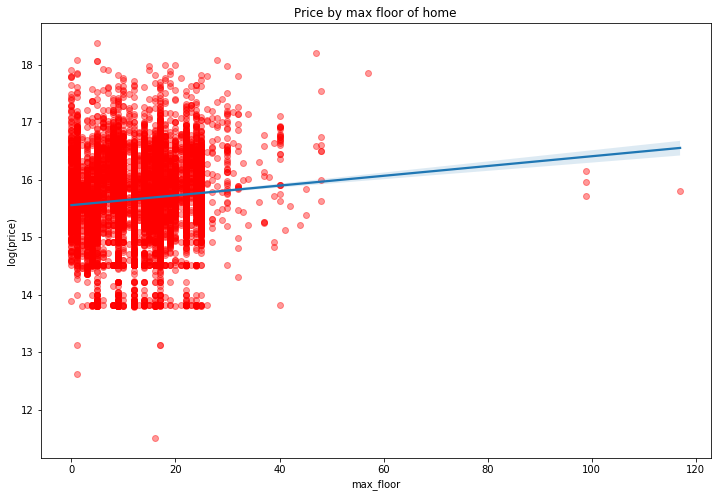Again a small positive correlation. This effect however is likely being confounded by the fact that the urban core has both more expensive real estate and taller buildings. So the height of the building alone is likely not what is determing price here.

In :
f, ax = plt.subplots(figsize=(12, 8))
plt.scatter(x=train_df['floor'], y=train_df['max_floor'], c='r', alpha=0.4)
plt.plot([0, 80], [0, 80], color='.5')

Out:
[<matplotlib.lines.Line2D at 0x19bd0da0>]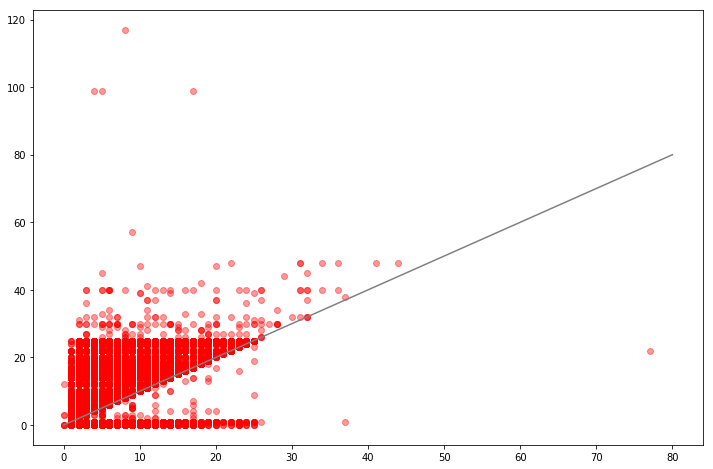The observations below the grey identity line have a floor greater than the number of floors in the building. That’s not good. How many are there?

In :
train_df.loc[train_df['max_floor'] < train_df['floor'], ['id', 'floor','max_floor']].head(20)

Out:
id floor max_floor
8216 8219 13.0 0.0
8268 8271 3.0 1.0
8499 8502 2.0 0.0
8531 8534 7.0 0.0
8912 8915 5.0 0.0
9161 9164 8.0 3.0
9257 9260 8.0 1.0
9309 9312 5.0 1.0
9388 9391 10.0 1.0
9412 9415 4.0 1.0
9423 9426 8.0 0.0
9442 9445 9.0 1.0
9452 9455 8.0 1.0
9482 9485 12.0 1.0
9561 9564 7.0 1.0
9689 9692 2.0 1.0
9696 9699 2.0 1.0
9724 9727 7.0 1.0
9764 9767 24.0 1.0
9822 9825 14.0 1.0

There are 1,493 observations where this is the case.

Demographic Characteristics

Now let’s move beyond the internal home characteristics and take a look at some of the basic demographic and geographic characteristics. First, the correlation plot.

In :
demo_feats = ['area_m', 'raion_popul', 'full_all', 'male_f', 'female_f', 'young_all', 'young_female',
'work_all', 'work_male', 'work_female', 'price_doc']
corrmat = train_df[demo_feats].corr()

In :
f, ax = plt.subplots(figsize=(10, 7))
plt.xticks(rotation='90')
sn.heatmap(corrmat, square=True, linewidths=.5, annot=True)

Out:
<matplotlib.axes._subplots.AxesSubplot at 0x1a4f9278>